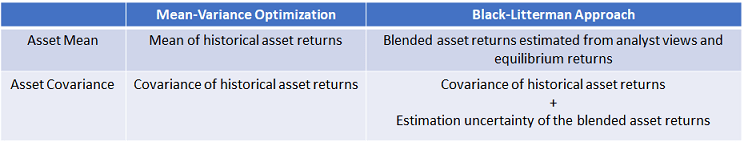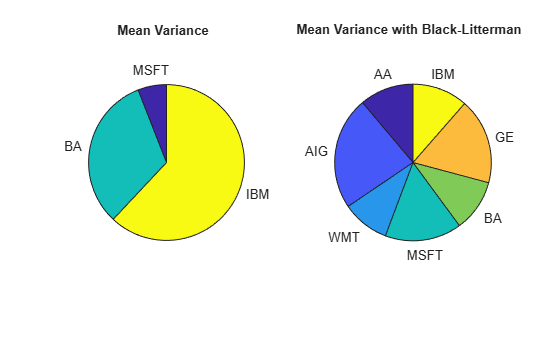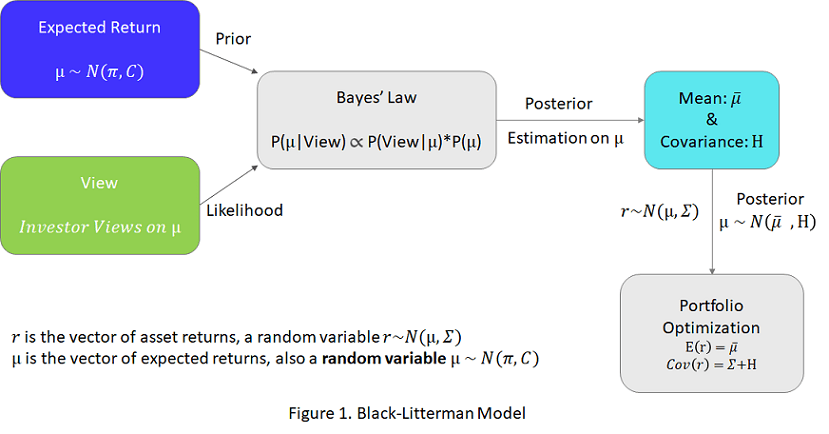Documentation

### This is machine translation

Mouseover text to see original. Click the button below to return to the English version of the page.

Note: This page has been translated by MathWorks. Click here to see
To view all translated materials including this page, select Country from the country navigator on the bottom of this page.

## Black-Litterman Portfolio Optimization

This example shows the workflow to implement the Black-Litterman model with the `Portfolio` class. The Black-Litterman model is an asset allocation approach that allows investment analysts to incorporate subjective views (based on investment analyst estimates) into market equilibrium returns. By blending analyst views and equilibrium returns instead of relying only on historical asset returns, the Black-Litterman model provides a systematic way to estimate the mean and covariance of asset returns.In the Black-Litterman model, the blended expected return is $\stackrel{-}{\mu }={\left[{\mathit{P}}^{\prime }{\Omega }^{-1}\mathit{P}+{\mathit{C}}^{-1}\right]}^{-1}\left[{\mathit{P}}^{\prime }{\Omega }^{-1}\mathit{q}+{\mathit{C}}^{-1}\pi \right]$ and the estimation uncertainty is $\mathrm{cov}\left(\mu \right)={\left[{\mathit{P}}^{\prime }{\Omega }^{-1}\mathit{P}+{\mathit{C}}^{-1}\right]}^{-1}$. To use the Black-Litterman model, you must prepare the inputs: $\mathit{P},\mathit{q}\text{\hspace{0.17em}},\Omega \text{\hspace{0.17em}},\text{\hspace{0.17em}}\pi ,$ and $\mathit{C}$. The inputs for $\mathit{P},\text{\hspace{0.17em}}\mathit{q}\text{\hspace{0.17em}},\text{\hspace{0.17em}}$and $\Omega$ are view-related and defined by the investment analyst. $\pi$ is the equilibrium return and $\mathit{C}$ is the uncertainty in prior belief. This example guides you to define these inputs and use the resulting blended returns in a portfolio optimization. For more information on the concept and derivation of the Black-Litterman model, see the Appendix section "Black-Litterman Model under a Bayesian Framework."

### Determine the Universe of Assets

The `dowPortfolio.xlsx` data set includes thirty assets and 1 benchmark. Seven assets from this data set comprise the investment universe in this example. The risk-free rate is assumed to be zero.

`T = readtable('dowPortfolio.xlsx');`

Define the asset universe and extract the asset returns from the price data.

```assetNames = ["AA", "AIG", "WMT", "MSFT", "BA", "GE", "IBM"]; benchmarkName = "DJI"; head(T(:,["Dates" benchmarkName assetNames]))```
```ans=8×9 table Dates DJI AA AIG WMT MSFT BA GE IBM ___________ _____ _____ _____ _____ _____ _____ _____ _____ 03-Jan-2006 10847 28.72 68.41 44.9 26.19 68.63 33.6 80.13 04-Jan-2006 10880 28.89 68.51 44.99 26.32 69.34 33.56 80.03 05-Jan-2006 10882 29.12 68.6 44.38 26.34 68.53 33.47 80.56 06-Jan-2006 10959 29.02 68.89 44.56 26.26 67.57 33.7 82.96 09-Jan-2006 11012 29.37 68.57 44.4 26.21 67.01 33.61 81.76 10-Jan-2006 11012 28.44 69.18 44.54 26.35 67.33 33.43 82.1 11-Jan-2006 11043 28.05 69.6 45.23 26.63 68.3 33.66 82.19 12-Jan-2006 10962 27.68 69.04 44.43 26.48 67.9 33.25 81.61 ```
```retnsT = tick2ret(T(:, 2:end)); assetRetns = retnsT(:, assetNames); benchRetn = retnsT(:, "DJI"); numAssets = size(assetRetns, 2);```

### Specify Views of the Market

The views represent the subjective views of the investment analyst regarding future market changes, expressed as $\mathbit{q}\text{\hspace{0.17em}}=\mathbit{P}*\mu \text{\hspace{0.17em}}+\text{\hspace{0.17em}}\epsilon ,\text{\hspace{0.17em}\hspace{0.17em}}\text{\hspace{0.17em}}\epsilon ~\mathit{N}\left(0,\text{\hspace{0.17em}}\Omega \right),\text{\hspace{0.17em}}\Omega =\mathrm{diag}\left({\omega }_{1},{\omega }_{2},...{\omega }_{\mathit{v}}\right)$, where v is total number of views. For more information, see the Appendix section "Assumptions and Views." With v views and k assets, $\mathit{P}$ is a v-by-k matrix, $\mathbit{q}$ is a v-by-1 vector, and $\Omega$ is a v-by-v diagonal matrix (representing the independent uncertainty in the views). The views do not necessarily need to be independent and the structure of $\Omega$ can be chosen to account for investment analyst uncertainties in the views . The smaller the ${\omega }_{\mathit{i}}$ in $\Omega$, the smaller the variance in the distribution of the ith view's uncertainty, and the stronger the investor's ith view. This example assumes three independent views.

1. Weak view that predicts AIG is going to have 5% annual return with uncertainty 1e-3.

2. Weak view that predicts WMT is going to have 3% annual return with uncertainty 1e-3.

3. Strong view that predicts MSFT is going to outperform IBM by 5% annual return with uncertainty 1e-5.

```v = 3; % total 3 views P = zeros(v, numAssets); q = zeros(v, 1); Omega = zeros(v); % View 1 P(1, assetNames=="AIG") = 1; q(1) = 0.05; Omega(1, 1) = 1e-3; % View 2 P(2, assetNames=="WMT") = 1; q(2) = 0.03; Omega(2, 2) = 1e-3; % View 3 P(3, assetNames=="MSFT") = 1; P(3, assetNames=="IBM") = -1; q(3) = 0.05; Omega(3, 3) = 1e-5;```

Visualize the three views in table form.

`viewTable = array2table([P q diag(Omega)], 'VariableNames', [assetNames "View_Return" "View_Uncertainty"]) `
```viewTable=3×9 table AA AIG WMT MSFT BA GE IBM View_Return View_Uncertainty __ ___ ___ ____ __ __ ___ ___________ ________________ 0 1 0 0 0 0 0 0.05 0.001 0 0 1 0 0 0 0 0.03 0.001 0 0 0 1 0 0 -1 0.05 1e-05 ```

Because the returns from `dowPortfolio.xlsx` data set are daily returns and the views are on the annual returns, you must convert views to be on daily returns.

```q = q/252; Omega = Omega/252;```

### Determine the Covariance from the Historical Asset Returns

$\Sigma$ is the covariance of the historical asset returns.

`Sigma = cov(assetRetns.Variables);`

### Determine the Uncertainty C

The Black-Litterman model makes the assumption that the structure of $\mathit{C}$ is proportional to the covariance $\Sigma$. Therefore, $\mathit{C}=\tau \Sigma$, where $\tau$ is a small constant. A smaller $\tau$ indicates a higher confidence in the prior belief of $\mu$. The work of He and Litterman uses a value of 0.025. Other authors suggest using 1/n where n is the number of data points used to generate the covariance matrix . This example uses 1/n.

```tau = 1/size(assetRetns.Variables, 1); C = tau*Sigma;```

### Market Implied Equilibrium Return

In the absence of any views, the equilibrium returns are likely equal to the implied returns from the equilibrium portfolio holding. In practice, the applicable equilibrium portfolio holding can be any optimal portfolio that the investment analyst would use in the absence of additional views on the market, such as the portfolio benchmark, an index, or even the current portfolio . In this example, you use linear regression to find a market portfolio that tracks the returns of the DJI benchmark. Then, you use the market portfolio as the equilibrium portfolio and the equilibrium returns are implied from the market portfolio. The Local Function `findMarketPortfolioAndImpliedReturn`, defined at the end of this example, implements the equilibrium returns. This function takes historical asset returns and benchmark returns as inputs and outputs the market portfolio and the corresponding implied returns.

`[wtsMarket, PI] = findMarketPortfolioAndImpliedReturn(assetRetns.Variables, benchRetn.Variables);`

### Compute the Estimated Mean Return and Covariance

Use the $\mathit{P},\text{\hspace{0.17em}}\mathit{q}\text{\hspace{0.17em}},\text{\hspace{0.17em}}\Omega \text{\hspace{0.17em}},\text{\hspace{0.17em}}\pi \text{\hspace{0.17em}},$ and $\mathit{C}$ inputs to compute the blended asset return and variance using the Black-Litterman model.

You can compute $\stackrel{-}{\mu }$ and $\mathrm{cov}\left(\mu \right)$ directly by using this matrix operation:

$\stackrel{-}{\mu }={\left[{\mathit{P}}^{\prime }{\Omega }^{-1}\mathit{P}+{\mathit{C}}^{-1}\right]}^{-1}\left[{\mathit{P}}^{\prime }{\Omega }^{-1}\mathit{q}+{\mathit{C}}^{-1}\pi \right]$,$\text{\hspace{0.17em}}\mathrm{cov}\left(\mu \right)={\left[{\mathit{P}}^{\prime }{\Omega }^{-1}\mathit{P}+{\mathit{C}}^{-1}\right]}^{-1}$

```mu_bl = (P'*(Omega\P) + inv(C)) \ ( C\PI + P'*(Omega\q)); cov_mu = inv(P'*(Omega\P) + inv(C));```

Comparing the blended expected return from Black-Litterman model to the prior belief of expected return $\pi$, you find that the expected return from Black-Litterman model is indeed a mixture of both prior belief and investor views. For example, as shown in the table below, the prior belief assumes similar returns for MSFT and IBM, but in the blended expected return, MSFT has a higher return than IBM by more than 4%. This difference is due to the imposed strong view that MSFT outperforms IBM by 5%.

```table(assetNames', PI*252, mu_bl*252, 'VariableNames', ["Asset_Name", ... "Prior_Belief_of_Expected_Return", "Black_Litterman_Blended_Expected_Return"])```
```ans=7×3 table Asset_Name Prior_Belief_of_Expected_Return Black_Litterman_Blended_Expected_Return __________ _______________________________ _______________________________________ "AA" 0.19143 0.19012 "AIG" 0.14432 0.13303 "WMT" 0.15754 0.1408 "MSFT" 0.14071 0.17557 "BA" 0.21108 0.2017 "GE" 0.13323 0.12525 "IBM" 0.14816 0.12877 ```

### Portfolio Optimization and Results

The `Portfolio` object in Financial Toolbox™ implements the Markowitz mean variance portfolio optimization framework. Using a `Portfolio` object, you can find the efficient portfolio for a given risk or return level, and you can also maximize the Sharpe ratio.

Use `estimateMaxSharpeRatio` with the `Portfolio` object to find allocations with the maximum Sharpe ratio for the following portfolios:

• Portfolio with asset mean and covariance from historical asset returns

• Portfolio with blended asset return and covariance from the Black-Litterman model

```port = Portfolio('NumAssets', numAssets, 'lb', 0, 'budget', 1, 'Name', 'Mean Variance'); port = setAssetMoments(port, mean(assetRetns.Variables), Sigma); wts = estimateMaxSharpeRatio(port); portBL = Portfolio('NumAssets', numAssets, 'lb', 0, 'budget', 1, 'Name', 'Mean Variance with Black-Litterman'); portBL = setAssetMoments(portBL, mu_bl, Sigma + cov_mu); wtsBL = estimateMaxSharpeRatio(portBL); ax1 = subplot(1,2,1); idx = wts>0.001; pie(ax1, wts(idx), assetNames(idx)); title(ax1, port.Name ,'Position', [-0.05, 1.6, 0]); ax2 = subplot(1,2,2); idx_BL = wtsBL>0.001; pie(ax2, wtsBL(idx_BL), assetNames(idx_BL)); title(ax2, portBL.Name ,'Position', [-0.05, 1.6, 0]);``````table(assetNames', wts, wtsBL, 'VariableNames', ["AssetName", "Mean_Variance", ... "Mean_Variance_with_Black_Litterman"])```
```ans=7×3 table AssetName Mean_Variance Mean_Variance_with_Black_Litterman _________ _____________ __________________________________ "AA" 6.5494e-11 0.1115 "AIG" 6.6964e-12 0.23314 "WMT" 2.6063e-12 0.098048 "MSFT" 0.059393 0.15824 "BA" 0.32068 0.10748 "GE" 7.3366e-10 0.1772 "IBM" 0.61993 0.11439 ```

When you use the values for the blended asset return and the covariance from the Black-Litterman model in a mean-variance optimization, the optimal allocations reflect the views of the investment analyst directly. The allocation from the Black-Litterman model is more diversified, as the pie chart shows. Also, the weights among the assets in the Black-Litterman model agree with the investment analyst views. For example, when you compare the Black-Litterman result with the plain mean-variance optimization result, you can see that the Black-Litterman result is more heavily invested in MSFT than in IBM. This is because the investment analyst has a strong view that MSFT will outperform IBM.

### Local Functions

```function [wtsMarket, PI] = findMarketPortfolioAndImpliedReturn(assetRetn, benchRetn) % Find the market portfolio that tracks the benchmark and its corresponding implied expected return.```

The implied return is calculated by reverse optimization. The risk-free rate is assumed to be zero. The general formulation of a portfolio optimization is given by the Markowitz optimization problem: $\underset{\omega }{\mathrm{arg}\mathrm{max}}\phantom{\rule{0.16666666666666666em}{0ex}}\phantom{\rule{0.16666666666666666em}{0ex}}\phantom{\rule{0.16666666666666666em}{0ex}}{\omega }^{T}\mu -\frac{\delta }{2}{\omega }^{T}\Sigma \omega$. Here $\omega$ is an N-element vector of asset weights, $\mu$ is an N-element vector of expected asset returns, $\Sigma$ is the N-by-N covariance matrix of asset returns, and $\delta$ is a positive risk aversion parameter. Given $\delta \text{\hspace{0.17em}}$, in the absence of constraints, a closed form solution to this problem is $\omega =\frac{1}{\delta }{\Sigma }^{-1}\mu$. Therefore, with a market portfolio, the implied expected return is $\pi =\delta \Sigma {\omega }_{mkt}$. For more information on computing implied return with constraints (for example, a budget constraint), refer to Herold's work .

To compute an implied expected return, you need $\Sigma ,\phantom{\rule{0.5em}{0ex}}{\omega }_{mkt},\phantom{\rule{0.5em}{0ex}}\delta$.

1) Find $\Sigma$.

$\Sigma$ is calculated from historical asset returns.

`Sigma = cov(assetRetn);`

2) Find the market portfolio.

To find the market portfolio, regress against the DJI. The imposed constraints are fully invested and long only: $\sum _{i=1}^{n}{\omega }_{i}=1,0\le {\omega }_{i},\phantom{\rule{0.16666666666666666em}{0ex}}\phantom{\rule{0.16666666666666666em}{0ex}}\phantom{\rule{0.16666666666666666em}{0ex}}\forall i\in \left\{1,...,n\right\}$

```numAssets = size(assetRetn,2); LB = zeros(1,numAssets); Aeq = ones(1,numAssets); Beq = 1; opts = optimoptions('lsqlin','Algorithm','interior-point', 'Display',"off"); wtsMarket = lsqlin(assetRetn, benchRetn, [], [], Aeq, Beq, LB, [], [], opts);```

3) Find $\delta$.

Multiply both sides of $\pi =\delta \Sigma {\omega }_{mkt}$ with ${\omega }_{mkt}^{T}$ to output $\delta =\frac{SharpeRatio}{{\sigma }_{m}}$. Here, the Benchmark is assumed to be the Sharpe ratio maximizing and this is used as market Sharpe ratio. Alternatively, you can calibrate an annualized Sharpe ratio to be 0.5, which leads to `shpr`=0.5/`sqrt`(252) . ${\sigma }_{m}$ is the standard deviation of the market portfolio.

```shpr = mean(benchRetn)/std(benchRetn); delta = shpr/sqrt(wtsMarket'*Sigma*wtsMarket); ```

4) Compute the implied expected return.

Assuming that the market portfolio maximizes the Sharpe ratio, the implied return, without the effects from constraints, is computed directly as $\pi =\delta \Sigma \omega$ .

```PI = delta*Sigma*wtsMarket; end```

### Assumptions and Views

Assume that the investment universe is composed of k assets and the vector of asset returns $\mathbit{r}$ is modeled as a random variable, following a multivariate normal distribution $\mathbit{r}~\mathit{N}\left(\mu ,\Sigma \right)$. $\Sigma$ is the covariance from historical asset returns. The unknown model parameter is the expected return $\mu$. From the perspective of Bayesian statistics, the Black-Litterman model attempts to estimate $\mu$ by combining the investment analyst views (or "observations of the future") and some prior knowledge about $\mu$.

In addition, assume the prior knowledge that $\mu$ is a normally distributed random variable $\mu ~\mathit{N}\left(\pi ,\mathbit{C}\right)$ [1, 2]. In the absence of any views (observations), the prior mean $\pi$ is likely to be the equilibrium returns, implied from the equilibrium portfolio holding. In practice, the applicable equilibrium portfolio holding is not necessarily the equilibrium portfolio, but rather a target optimal portfolio that the investment analyst would use in the absence of additional views on the market, such as the portfolio benchmark, an index, or even the current portfolio. $\mathit{C}$ represents the uncertainty in the prior and the Black-Litterman model makes the assumption that the structure of$\text{\hspace{0.17em}}\mathit{C}$ is $\tau \Sigma$. $\tau$ is a small constant, and many authors use different values. A detailed discussion about $\tau$ can be found in .

Observations are necessary to perform a statistical inference on $\mu$. In the Black-Litterman model, the observations are views about future asset returns expressed at the portfolio level. A view is the expected return of a portfolio composed of the universe of k assets. Usually, the portfolio return has uncertainty, so an error term is added to catch the departure. Assume that there is a total of v views. For a view $\mathit{i}$, ${\mathbit{p}}_{\mathit{i}}$ is a row vector with dimension 1 x k, and ${\mathit{q}}_{\mathit{i}}$ is a scalar .

${\mathit{q}}_{\mathit{i}}$ = $Ε\left[{\mathbit{p}}_{\mathit{i}}*\mathbit{r}\text{\hspace{0.17em}}|\text{\hspace{0.17em}}\mu \right]+{\epsilon }_{\mathit{i}\text{\hspace{0.17em}}}\text{\hspace{0.17em}},\text{\hspace{0.17em}}\text{\hspace{0.17em}}\mathit{i}=1,2,...,\mathit{v}$

You can stack the v views vertically, and $\Omega$ is the covariance of the uncertainties from all views. Assume that the uncertainties are independent.

$\mathbit{q}\text{\hspace{0.17em}}=\text{\hspace{0.17em}}Ε\left[\mathbit{P}*\mathbit{r}\text{\hspace{0.17em}}|\text{\hspace{0.17em}}\mu \right]+\text{\hspace{0.17em}}\epsilon ,\text{\hspace{0.17em}}\text{\hspace{0.17em}}\epsilon ~\mathit{N}\left(0,\text{\hspace{0.17em}}\Omega \right),\text{\hspace{0.17em}}\text{\hspace{0.17em}}\Omega =\mathrm{diag}\left({\omega }_{1},{\omega }_{2},...{\omega }_{\mathit{v}}\right)$.

Note that $\Omega$ does not necessarily need to be a diagonal matrix. The investment analyst can choose the structure of $\Omega$ to account for their uncertainties in the views .

Under the previous assumption $\mathbit{r}~\mathit{N}\left(\mu ,\Sigma \right)$, it follows that

$\mathbit{q}\text{\hspace{0.17em}}=\mathbit{P}*\mu \text{\hspace{0.17em}}+\text{\hspace{0.17em}}\epsilon ,\text{\hspace{0.17em}\hspace{0.17em}}\text{\hspace{0.17em}}\epsilon ~\mathit{N}\left(0,\text{\hspace{0.17em}}\Omega \right),\text{\hspace{0.17em}}\Omega =\mathrm{diag}\left({\omega }_{1},{\omega }_{2},...{\omega }_{\mathit{v}}\right)$.

### The Bayesian Definition of the Black-Litterman Model

Based on Bayesian statistics, it is known that:$\text{\hspace{0.17em}}\mathrm{posterior}\propto \mathrm{likelihood}*\mathrm{prior}$.In the context of the Black-Litterman model,$\text{\hspace{0.17em}}\mathrm{posterior}\propto \mathrm{likelihood}*\mathrm{prior}$ is expressed as $\mathit{f}\left(\mu |\mathit{q}\right)$$\propto$ $\mathit{f}\left(\mathit{q}|\mu \right)$*$\mathit{f}\left(\mu \right),$ where each Bayesian term is defined as follows :

• The likelihood is how likely it is for the views to happen given $\mu$ and is expressed as $\mathit{f}\left(\mathit{q}|\mu \right)\propto \mathrm{exp}\left[-\frac{1}{2}{\left(\mathit{P}\mu -\mathit{q}\right)}^{\prime }{\Omega }^{-1}\left(\mathit{P}\mu -\mathit{q}\right)\right].$

• The prior assumes the prior knowledge that $\mu ~\mathit{N}\left(\pi ,\mathit{C}\right)$ and is expressed as $\mathit{f}\left(\mu \right)\text{\hspace{0.17em}\hspace{0.17em}\hspace{0.17em}}$$\propto \mathrm{exp}\left[-\frac{1}{2}{\left(\mu -\pi \right)}^{\prime }{\mathit{C}}^{-1}\left(\mu -\pi \right)\right].$

• The posterior is the distribution of $\mu$ given views and is expressed as $\mathit{f}\left(\mu |\mathit{q}\right)\text{\hspace{0.17em}}\propto \mathrm{exp}\left[-\frac{1}{2}{\left(\mathit{P}\mu -\mathit{q}\right)}^{\prime }{\Omega }^{-1}\left(\mathit{P}\mu -\mathit{q}\right)-\frac{1}{2}{\left(\mu -\pi \right)}^{\prime }{\mathit{C}}^{-1}\left(\mu -\pi \right)\right].$

As previously stated, the posterior distribution of$\text{\hspace{0.17em}}\mu$ is also a normal distribution. By completing the squares, you can derive the posterior mean and covariance as $\stackrel{-}{\mu }={\left[{\mathit{P}}^{\mathit{T}}{\Omega }^{-1}\mathit{P}+{\mathit{C}}^{-1}\right]}^{-1}\left[{\mathit{P}}^{\mathit{T}}{\Omega }^{-1}\mathit{q}+{\mathit{C}}^{-1}\pi \right]$, $\mathrm{cov}\left(\mu \right)={\left[{\mathit{P}}^{\mathit{T}}{\Omega }^{-1}\mathit{P}+{\mathit{C}}^{-1}\right]}^{-1}$.

Finally, by combining the Bayesian posterior distribution of$\text{\hspace{0.17em}}\mu$ and the model of asset returns $\mathbit{r}~\mathit{N}\left(\mu ,\Sigma \right)$, you then have the posterior prediction of asset returns as $\mathbit{r}~\mathit{N}\left(\stackrel{-}{\mu },\text{\hspace{0.17em}}\Sigma +\mathrm{cov}\left(\mu \right)\right)$.

### References

1. Walters, J. "The Black-Litterman Model in Detail." 2014. Available at SSRN: https://ssrn.com/abstract=1314585.

2. Kolm, P. N., and Ritter, G. "On the Bayesian Interpretation of Black-Litterman." European Journal of Operational Research. Vol. 258, Number 2, 2017, pp. 564-572.

3. Walters, J. "A Discussion of $\tau$ (tau)." (http://www.blacklitterman.org/tau.html).

4. Attilio, M. "Beyond Black-Litterman in Practice: A Five-Step Recipe to Input Views on Non-Normal Markets." 2006. Available at SSRN: https://ssrn.com/abstract=872577 or http://dx.doi.org/10.2139/ssrn.872577.

5. Ulf, H. "Computing implied returns in a meaningful way." Journal of Asset Management. Vol 6, Number 1, 2005, pp. 53-64.

Download ebook